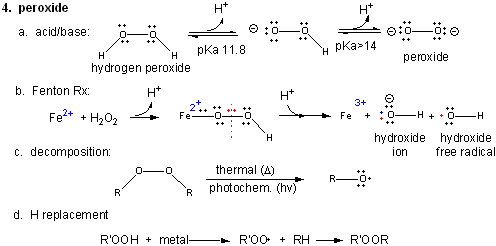# A3. The Reactions of Dioxygen and its Reduction Products

$$\newcommand{\vecs}{\overset { \rightharpoonup} {\mathbf{#1}} }$$ $$\newcommand{\vecd}{\overset{-\!-\!\rightharpoonup}{\vphantom{a}\smash {#1}}}$$$$\newcommand{\id}{\mathrm{id}}$$ $$\newcommand{\Span}{\mathrm{span}}$$ $$\newcommand{\kernel}{\mathrm{null}\,}$$ $$\newcommand{\range}{\mathrm{range}\,}$$ $$\newcommand{\RealPart}{\mathrm{Re}}$$ $$\newcommand{\ImaginaryPart}{\mathrm{Im}}$$ $$\newcommand{\Argument}{\mathrm{Arg}}$$ $$\newcommand{\norm}{\| #1 \|}$$ $$\newcommand{\inner}{\langle #1, #2 \rangle}$$ $$\newcommand{\Span}{\mathrm{span}}$$ $$\newcommand{\id}{\mathrm{id}}$$ $$\newcommand{\Span}{\mathrm{span}}$$ $$\newcommand{\kernel}{\mathrm{null}\,}$$ $$\newcommand{\range}{\mathrm{range}\,}$$ $$\newcommand{\RealPart}{\mathrm{Re}}$$ $$\newcommand{\ImaginaryPart}{\mathrm{Im}}$$ $$\newcommand{\Argument}{\mathrm{Arg}}$$ $$\newcommand{\norm}{\| #1 \|}$$ $$\newcommand{\inner}{\langle #1, #2 \rangle}$$ $$\newcommand{\Span}{\mathrm{span}}$$

1. Triplet O2 - Ground State:

Figure: Triplet O2 - Ground State• Metals ions - Metal ions are radicals themselves, so can react with dioxygen. Ex:
Fe2+ + O2 <--> [ Fe2+-- O2 <--> Fe3+-- O2-.] <--> Fe3+ + O2-. (superoxide)
• Autoxidation of organic molecules to produce peroxides. In this free radical reaction, several reactions occur, including
RH ---> R. (Initiation)
R. + O2 ---> ROO. (Propagation)
ROO. + RH ---> R. + ROOH (Propagation)
R. + R. ---> R--R (Termination)
ROO. + ROO. ---> ROOR + O2 (Termination)
ROO. + R. ---> ROOR (Termination)

The initiation step above occurs mostly at C atoms which can produce the most stable free radicals (allylic, benzylic position, and 3o > 2o >> 10 carbons). Hence unsaturated fatty acids are extra reactive at the methylene C that separate the double bonds.

Figure: unsaturated fatty acids are extra reactive at the methylene C that separate the double bonds2. Single O2 - Excited State.

Figure: Single O2 - Excited StateIt can be made from triplet oxygen by photoexcitation. Alternatively, it can be made from triplet oxygen through collision with an excited molecule which relaxes to the ground state after a radiationless transfer of energy to triplet oxygen to form reactive singlet oxygen. (This later process accounts for photobleaching of colored clothes when the conjugated dye molecules absorb UV and Vis light, relax by transferring energy to triplet oxygen to form singlet oxygen, which then chemically reacts with the conjugated double bonds in the dye. )

• Alkenes react with oxygen to form hydroperoxides, potentially through a epoxide intermediate
• Dienes reacts with oxygen in a Diels-Alder like reaction to form endoperoxides

3. Superoxide

Figure: Superoxide• Dismuation: O2-. + O2-. ---> H2O2 + O2
• Acid/Base: HO2. ----> O2-. + H+ (pKa = 4.8)
• With metal ions: Fe3+ (as in heme) + O2-. ---> O 2 + Fe2+

4. Peroxide

Figure: PeroxideIn contrast to dioxygen which contains multiple bonds between the O atoms, peroxide has only one bond. In fact, it is quite weak and requires only 38 kcal/mol to break it. Remember, bonds can be broken in a heterolytic way (both electrons in a bond go to one of the atoms, or in a homolytic fashion, in which the one electron goes to each atom.

• Acid/Base: H2O2 ---> HO2- ---> O22- (pKa1 = 11.8; pKa2 > 14)
• Reaction with Fe2+ - The Fenton Reaction: (similar to reaction of triplet O2 with Fe2+ above)
Fe2+ + OOH- <--> Fe2+-- OOH <--> Fe2+-- O + OH- <--> Fe3+-- O . <--> Fe3+ + OH. (last step a proton is added). In this reaction, a homolytic cleavage of the O--O bond occurs generating OH- and the hydroxy free radical, OH., which will react with any molecule it encounters.
• thermal or photochemical homolytic cleavage of peroxide. This forms alkoxide free radicals which reacts like the hydroxy free radical.
• Reactions with alkyl groups in the presence of metal ions such as Cu, Co, or Mn:
RH + R'OOH ----> ROOR'